STANDARDIZED RATES AND RATIOS Nigel Paneth MORTALITY RATES

• Slides: 34STANDARDIZED RATES AND RATIOS Nigel Paneth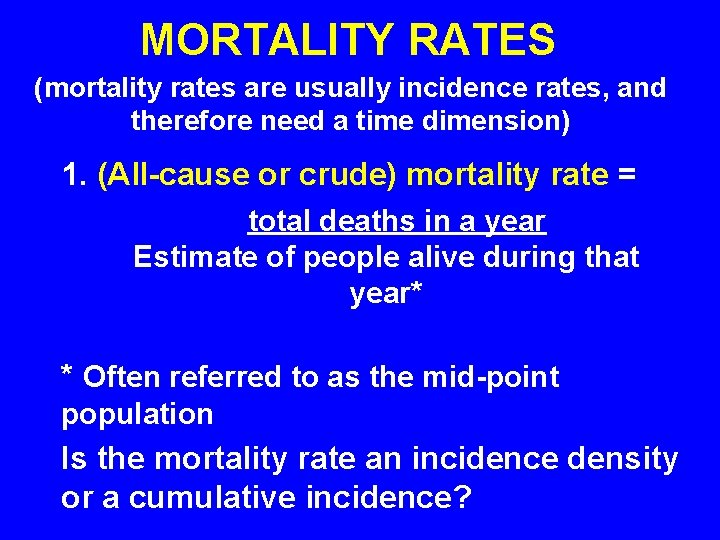MORTALITY RATES (mortality rates are usually incidence rates, and therefore need a time dimension) 1. (All-cause or crude) mortality rate = total deaths in a year Estimate of people alive during that year* * Often referred to as the mid-point population Is the mortality rate an incidence density or a cumulative incidence?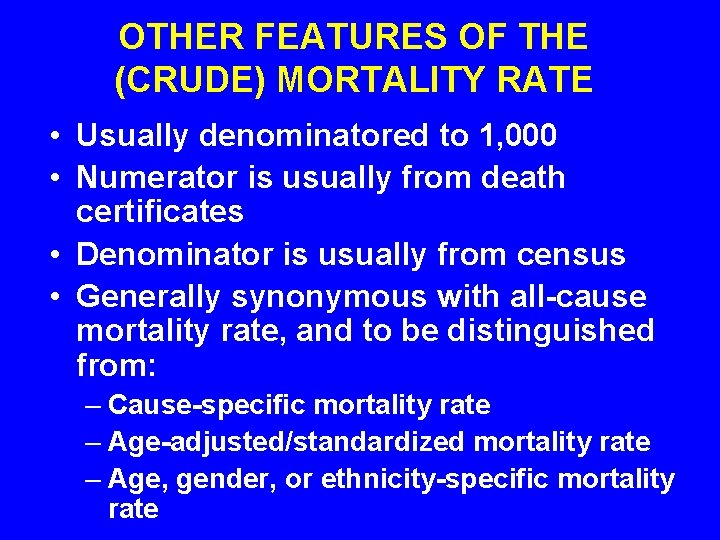OTHER FEATURES OF THE (CRUDE) MORTALITY RATE • Usually denominatored to 1, 000 • Numerator is usually from death certificates • Denominator is usually from census • Generally synonymous with all-cause mortality rate, and to be distinguished from: – Cause-specific mortality rate – Age-adjusted/standardized mortality rate – Age, gender, or ethnicity-specific mortality rate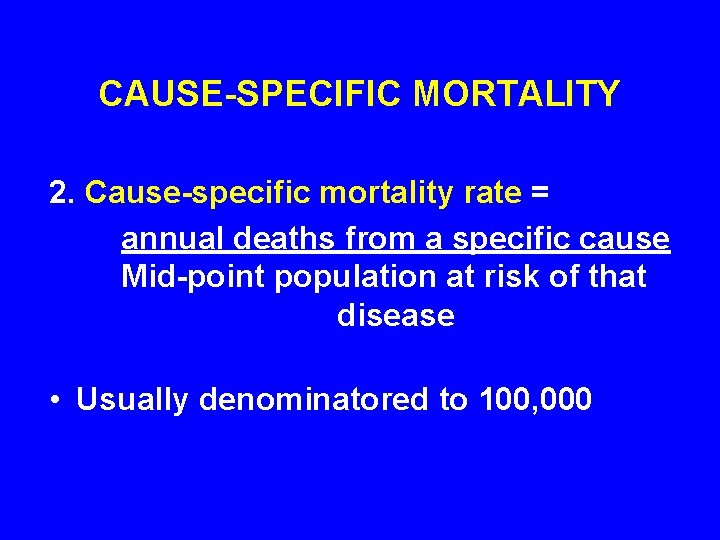CAUSE-SPECIFIC MORTALITY 2. Cause-specific mortality rate = annual deaths from a specific cause Mid-point population at risk of that disease • Usually denominatored to 100, 000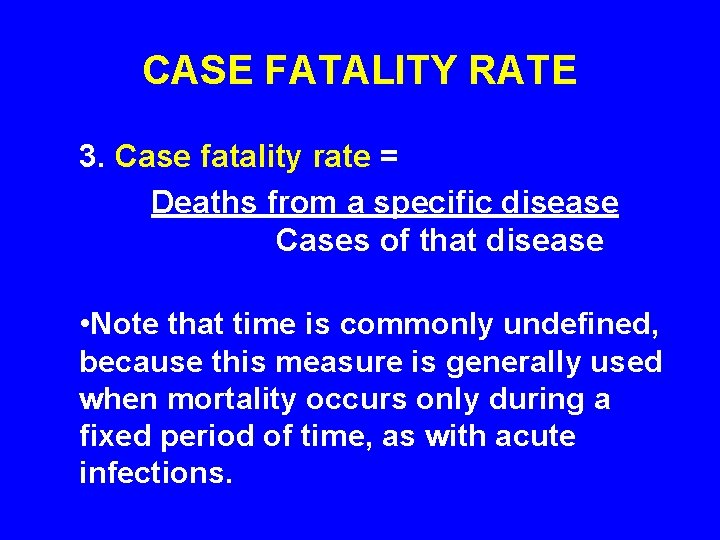CASE FATALITY RATE 3. Case fatality rate = Deaths from a specific disease Cases of that disease • Note that time is commonly undefined, because this measure is generally used when mortality occurs only during a fixed period of time, as with acute infections.Mortality Rates Cont’d 4. Proportionate mortality rate = Deaths from a specific cause Deaths from all causes • Note that this can be a misleading rate; Use with care, if at all. Almost all autopsy series base conclusions on proportionate mortality rate. Note that this is a proportion, and since it has no population denominator, is neither an incidence nor a prevalence rate.SURVIVAL RATES 5. Five-year survival rate = Number of people alive after five years Number alive at beginning of the interval • Commonly used in chronic diseases such as cancer, where mortality may be spread out over several years. Usually disease-specific. Any interval can be used, 10 years also fairly common.SPECIFIC MORTALITY RATES 6. Specific (or stratum-specific) mortality rate = A mortality rate in a specific segment of the population, such as 55 -60 year olds (agespecific), or in men (sex-specific) or in a population group (e. g. hispanic mortality rates) any other stratum of the population. Generally applied to all-cause mortality, though can be applied to cause-specific mortality as wellSTANDARDIZED MORTALITY RATES 7. Standardized (adjusted) rate = A rate which differs from a crude rate in having been standardized to a different population (usually to a standard population) to remove the influence of some extraneous variable, such as age.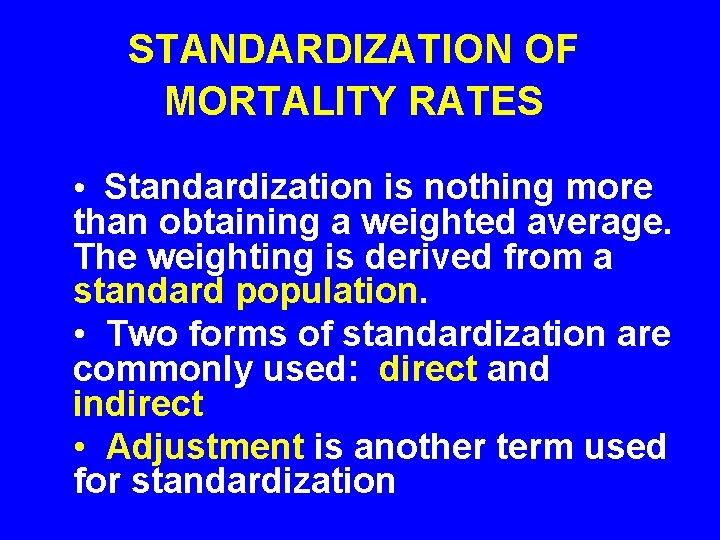STANDARDIZATION OF MORTALITY RATES • Standardization is nothing more than obtaining a weighted average. The weighting is derived from a standard population. • Two forms of standardization are commonly used: direct and indirect • Adjustment is another term used for standardizationAll forms of standardization involve first breaking down or decomposing a population’s mortality rate into two components: Component 1: The distribution of people in the population in groups (strata) having certain characteristics in common. For example, when we standardize for age, we often create strata of people of the same 10 -year age stratum (e. g. 25 -34 years, 35 -44 years, etc). We call these stratum-specific proportions. Component 2: The mortality rates in each of the strata. We call these stratum-specific mortality rates. For example, the mortality for 25 -34 year olds.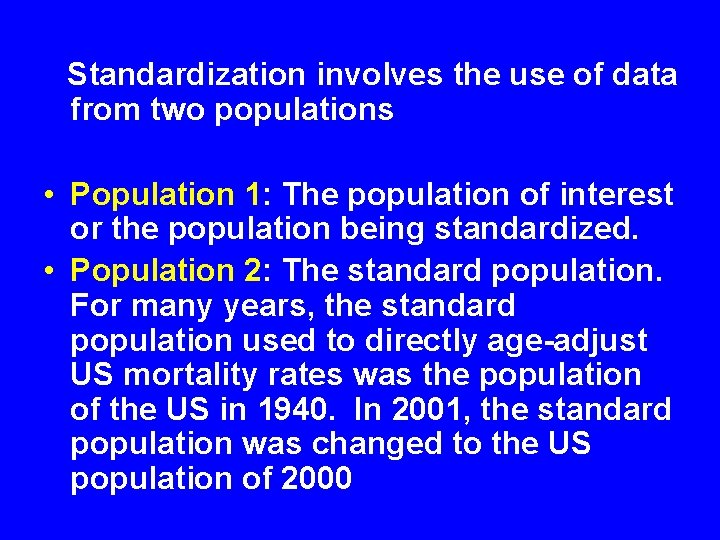Standardization involves the use of data from two populations • Population 1: The population of interest or the population being standardized. • Population 2: The standard population. For many years, the standard population used to directly age-adjust US mortality rates was the population of the US in 1940. In 2001, the standard population was changed to the US population of 2000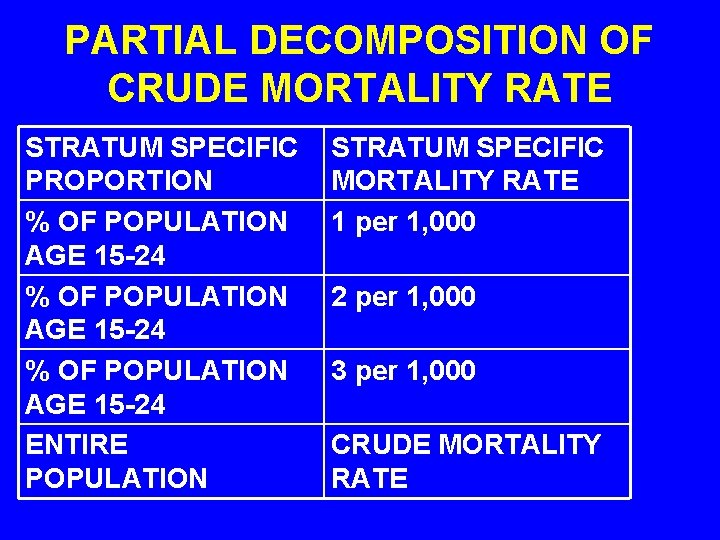PARTIAL DECOMPOSITION OF CRUDE MORTALITY RATE STRATUM SPECIFIC PROPORTION % OF POPULATION AGE 15 -24 ENTIRE POPULATION STRATUM SPECIFIC MORTALITY RATE 1 per 1, 000 2 per 1, 000 3 per 1, 000 CRUDE MORTALITY RATE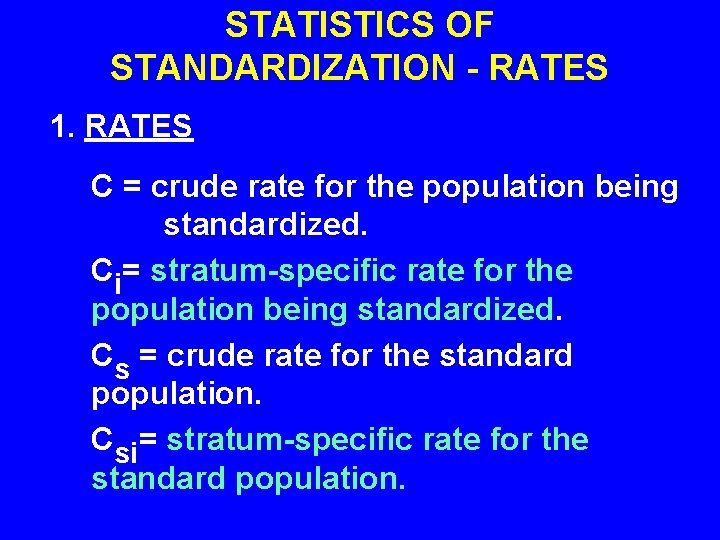STATISTICS OF STANDARDIZATION - RATES 1. RATES C = crude rate for the population being standardized. Ci= stratum-specific rate for the population being standardized. Cs = crude rate for the standard population. Csi= stratum-specific rate for the standard population.STATISTICS OF STANDARDIZATION - PROPORTIONS 2. PROPORTIONS Pi = Stratum-specific proportion in the population being standardized Psi = Stratum-specific proportion in the standard populationPRODUCTS OF STANDARDIZATION Cdirect = directly standardized rate. Cindirect = indirectly standardized rate.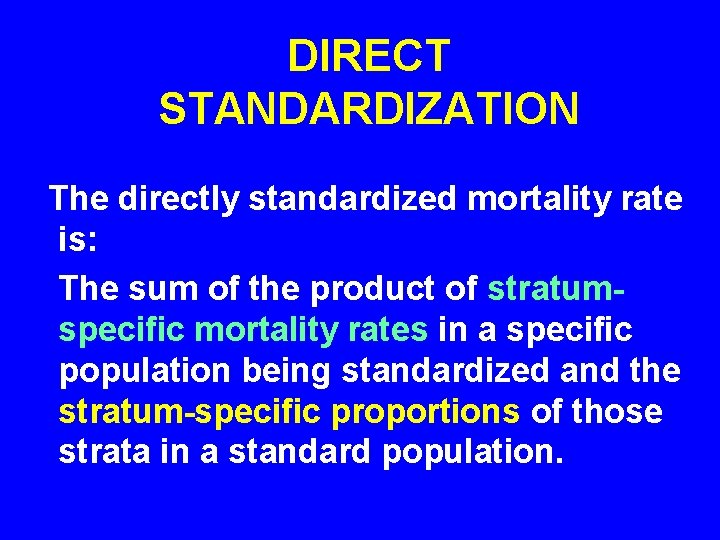DIRECT STANDARDIZATION The directly standardized mortality rate is: The sum of the product of stratumspecific mortality rates in a specific population being standardized and the stratum-specific proportions of those strata in a standard population.FORMULA FOR DIRECT STANDARDIZATION OF RATES Formula for direct standardization: i CDIRECT = (Ci x Psi) 0 The sum of the product of stratumspecific mortality rates in a specific population being standardized and the stratum- specific proportions of those strata in a standard population.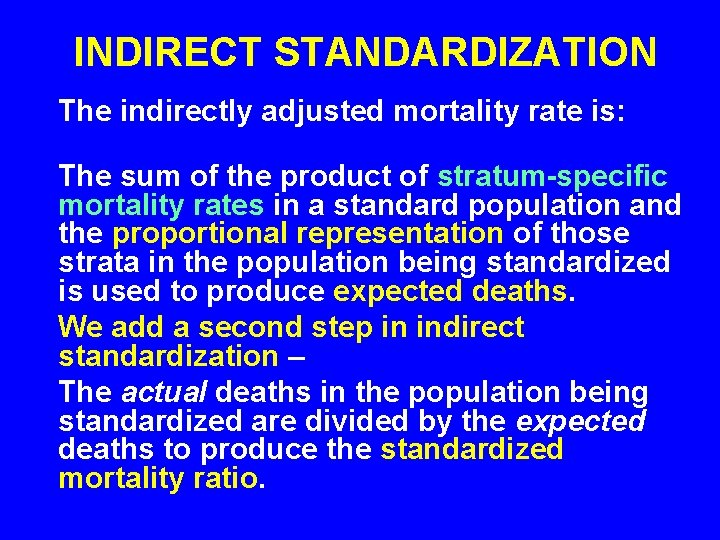INDIRECT STANDARDIZATION The indirectly adjusted mortality rate is: The sum of the product of stratum-specific mortality rates in a standard population and the proportional representation of those strata in the population being standardized is used to produce expected deaths. We add a second step in indirect standardization – The actual deaths in the population being standardized are divided by the expected deaths to produce the standardized mortality ratio.FORMULA FOR INDIRECT STANDARDIZATION CINDIRECT is calculated in two steps: 1. Calculate expected N of deaths in the population of interest: i ED = (Csi x Pi ) x 1, 000 0 2. Divide the actual deaths by the expected deaths (ED) to obtain the standardized mortality ratio (SMR). SMR = actual deaths/expected deathsCOMPARING STANDARDIZED MORTALITY RATES Direct standardization yields an expected rate (or standardized rate) which can then be compared to the crude rate, or to any other similarly standardized rate. Indirect standardization yields an expected number of deaths, which can then be compared to the number of actual deaths, as in the SMR, or to the expected number of deaths in another population.MNEMONIC DEVICE • When you use the MORTALITY RATES of the POPULATION OF INTEREST, you are DIRECTLY standardizing. • When you use the MORTALITY RATES of the STANDARD POPULATION, you are INDIRECTLY standardizing.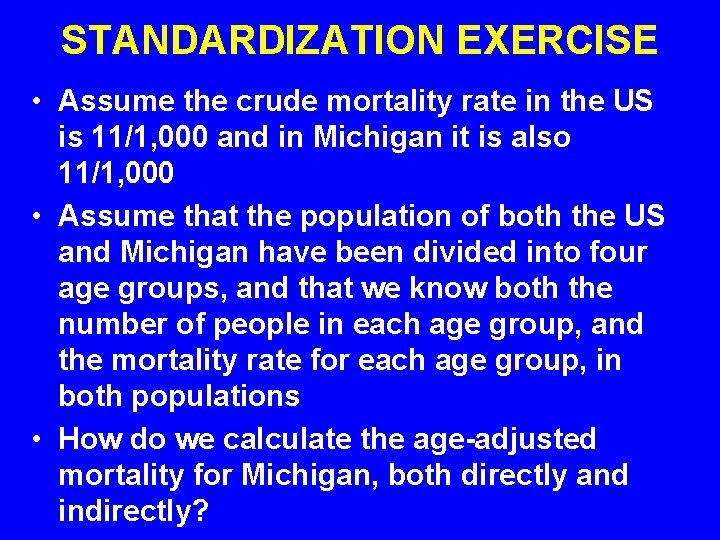STANDARDIZATION EXERCISE • Assume the crude mortality rate in the US is 11/1, 000 and in Michigan it is also 11/1, 000 • Assume that the population of both the US and Michigan have been divided into four age groups, and that we know both the number of people in each age group, and the mortality rate for each age group, in both populations • How do we calculate the age-adjusted mortality for Michigan, both directly and indirectly?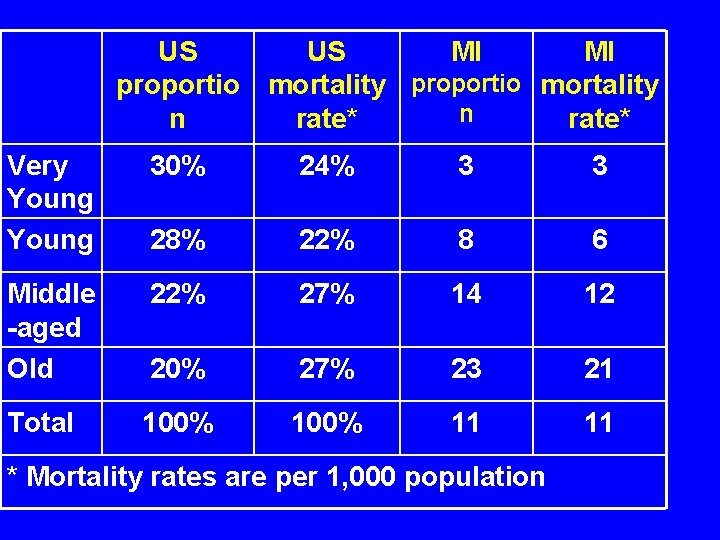US US MI MI proportio mortality n n rate* Very Young 30% 24% 3 3 28% 22% 8 6 Middle -aged Old 22% 27% 14 12 20% 27% 23 21 Total 100% 11 11 * Mortality rates are per 1, 000 populationA. To directly standardize, use the standard population distribution (the US), and the agespecific mortality rates for the population of interest (Michigan). Then calculate the mortality rate that would apply in Michigan if it had the same age distribution as the US. US POP MI RATE. 30 x 3/1, 000 = 0. 90/1, 000 +. 28 x 6/1, 000 = 1. 68/1, 000 +. 22 x 12/1, 000 = 2. 64/1, 000 +. 20 x 21/1, 000 = 4. 20/1, 000 + This sum adds up to the Age-standardized MI mortality rate of 9. 42/1, 000.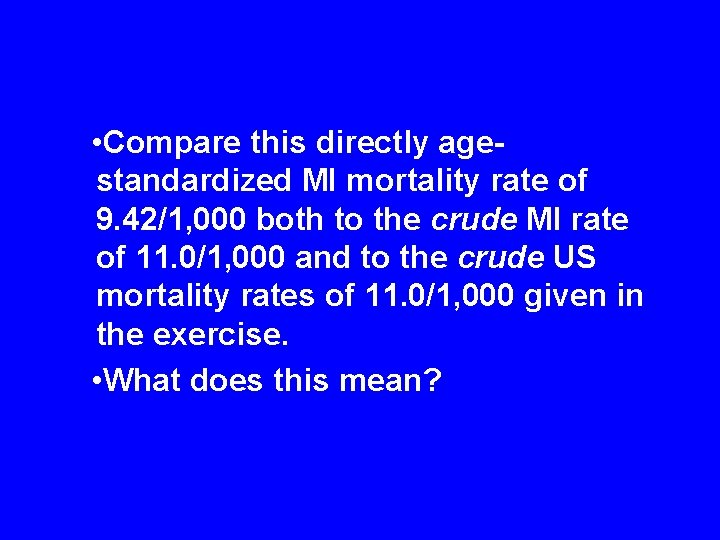• Compare this directly agestandardized MI mortality rate of 9. 42/1, 000 both to the crude MI rate of 11. 0/1, 000 and to the crude US mortality rates of 11. 0/1, 000 given in the exercise. • What does this mean?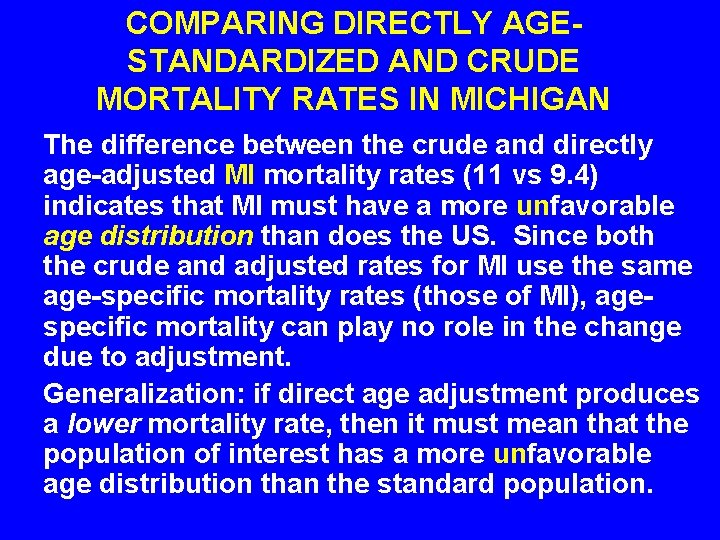COMPARING DIRECTLY AGESTANDARDIZED AND CRUDE MORTALITY RATES IN MICHIGAN The difference between the crude and directly age-adjusted MI mortality rates (11 vs 9. 4) indicates that MI must have a more unfavorable age distribution than does the US. Since both the crude and adjusted rates for MI use the same age-specific mortality rates (those of MI), agespecific mortality can play no role in the change due to adjustment. Generalization: if direct age adjustment produces a lower mortality rate, then it must mean that the population of interest has a more unfavorable age distribution than the standard population.COMPARING DIRECTLY AGESTANDARDIZED MI MORTALITY RATES TO US MORTALITY RATES The difference between the directly age- adjusted MI mortality and the crude US mortality indicates that MI has, on average, lower age-specific mortality rates. Both statistics have the same age distribution. Generalization: if direct age-adjustment produces a lower mortality rate in the population of interest, then it must mean that the standard population has a more unfavorable age-specific mortality.INDIRECT STANDARDIZATION To indirectly standardize, use the age distribution of the population of interest (Michigan) and the agespecific mortality rates of the standard population (the US) and calculate the expected number of deaths that would occur in Michigan, if the US age-specific mortality rates were to apply.INDIRECT STANDARDIZATION STEP 1 – CALCULATE EXPECTED DEATHS 1. Calculate the no. of expected deaths (ED). Assume a population of 1, 000 distributed as in Michigan, then MI POP US RATE 240 x 3/1, 000 = 0. 72 ED + 220 x 8/1, 000 = 1. 76 ED + 270 x 14/1, 000 = 3. 78 ED + 270 x 23/1, 000 = 6. 21 ED + This adds up to 12. 47 expected deaths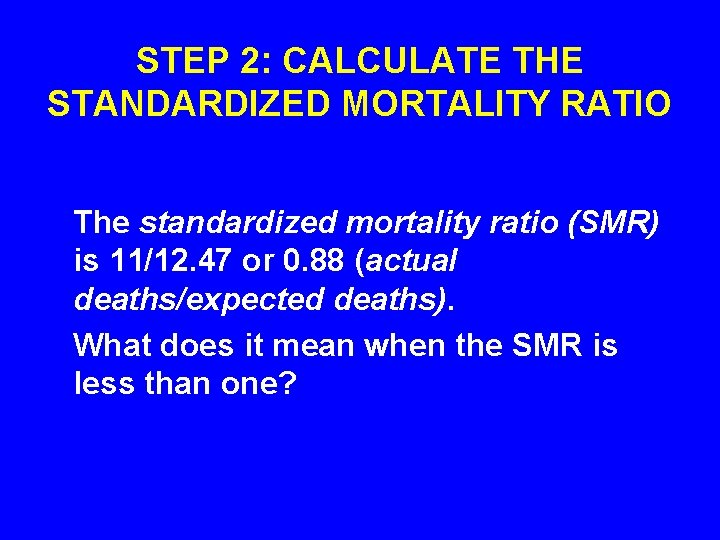STEP 2: CALCULATE THE STANDARDIZED MORTALITY RATIO The standardized mortality ratio (SMR) is 11/12. 47 or 0. 88 (actual deaths/expected deaths). What does it mean when the SMR is less than one?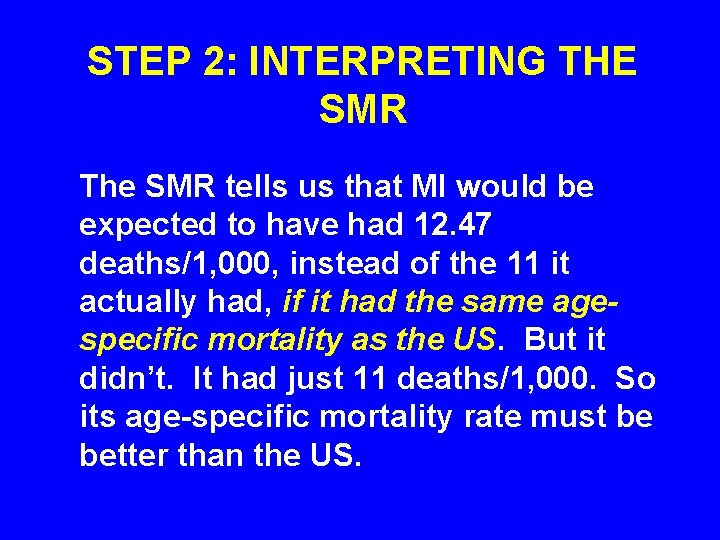STEP 2: INTERPRETING THE SMR The SMR tells us that MI would be expected to have had 12. 47 deaths/1, 000, instead of the 11 it actually had, if it had the same agespecific mortality as the US. But it didn’t. It had just 11 deaths/1, 000. So its age-specific mortality rate must be better than the US.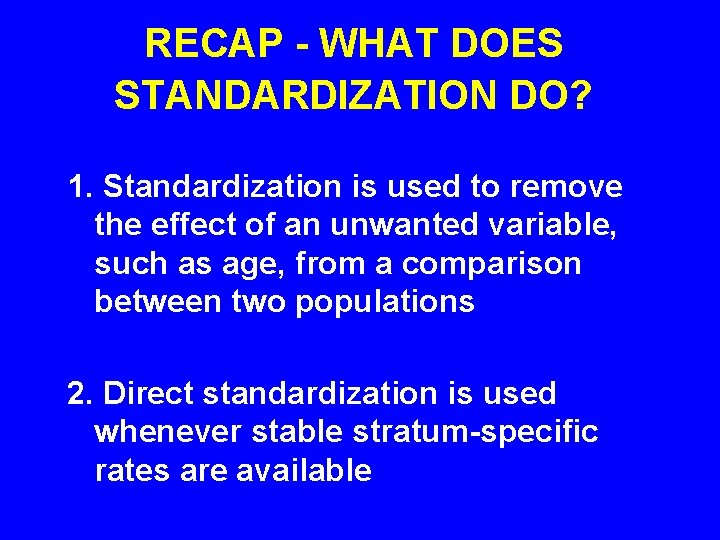RECAP - WHAT DOES STANDARDIZATION DO? 1. Standardization is used to remove the effect of an unwanted variable, such as age, from a comparison between two populations 2. Direct standardization is used whenever stable stratum-specific rates are available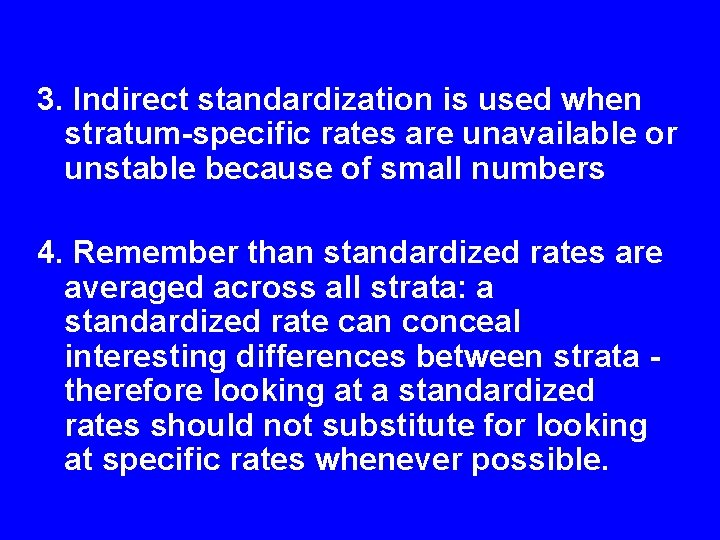3. Indirect standardization is used when stratum-specific rates are unavailable or unstable because of small numbers 4. Remember than standardized rates are averaged across all strata: a standardized rate can conceal interesting differences between strata - therefore looking at a standardized rates should not substitute for looking at specific rates whenever possible.